## How to use group actions

### Quick description

This article provides a list of different ways to study groups by their actions.

### General discussion

Click here for a brief discussion of group actions. ( Many groups arise naturally as groups of transformations of one kind or another. For example, the cyclic group of ordercan be thought of as the group of rotations of a regular-gon. But even a group that arises in a different way can often be thought of very fruitfully as a group of transformations: it's just that one has to find a mathematical object to be transformed.

More formally, if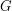is a group, and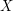is a set, then an action ofonis a homomorphism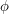fromto the set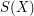of all bijections fromto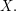That is, for each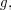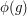is a bijection fromto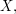and these bijections need to compose in a way that reflects the multiplication in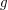: we need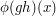to be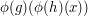for every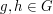and every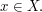If we are dealing with just one action, it can be nicer to write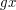instead of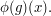That is, we think ofas actually equalling a bijection rather than being transformed into one. Then the rule is that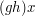should equal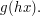(This is actually the definition of a left action. Analogously, we can write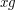for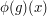, in which case we require that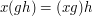. This is the definition of a right action.) Note that using the word "homomorphism" rather than "isomorphism" is intentional here: perhaps surprisingly, actions are often very useful even if different elements ofhave the same effect onSometimes the setis just a finite set, in which case a bijection fromtois naturally thought of as a permutation, and the action ofas a homomorphism fromto the symmetric group onBut oftenhas more structure: it might be a vector space, or a topological space, say. Then the interesting actions are the ones where the bijections fromtoare the structure preserving ones: invertible linear maps in the case of vector spaces, and homeomorphisms in the case of topological spaces.
)

### The articles

• Proving results by letting a group act on a finite set Quick description ( This article discusses various ways of deducing facts about groups by choosing appropriate actions on finite sets. Sometimes the group itself acts, and sometimes another group acts on a set that is defined in terms of the first group.)

• To show that a group element is non-trivial, show that it has a non-trivial action or image Quick description ( A groupmay be defined so indirectly that it can be difficult to distinguish one group element from another; but often there are ways to mapto a more concrete group, or to makeact on a more concrete space. Using such concrete representations, it becomes easier to distinguish elements in.)

• Representation theory Quick description ( When the seton which a group acts is a vector space and the bijections are linear, we have what is called a representation of the group. Representations are a very powerful way of studying groups. They also have many other applications, and representation theory is regarded as a branch of mathematics in its own right.)

• Use topology to study your group Quick description ( If you want to study a group, then it can help to realizeas the fundamental group of a topological space. This works best whenis infinite and discrete, and especially ifis finitely presented and torsion-free.)